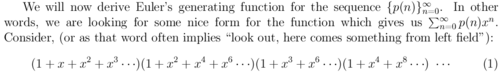Partition Function

5 = 5
5 = 4 + 1
5 = 3 + 2
5 = 3 + 1 + 1
5 = 2 + 2 + 1
5 = 2 + 1 + 1 + 1
5 = 1 + 1 + 1 + 1 + 1

There are 7 ways to split up five things. Seven different ways you could divide up 5 balls, 5 dolls, 5 wrapped-up candies; seven microstate subsets of a 5-molecule gas.How many ways are there to divide up 4 things? 8 things? 20,000 things? 198^198 things? Even if you had a few days to write a computer program that would brute-force count these things, how would you do it?

I would try to come up with a way to “break one off” from the largest number and count the “leaves” off each branch, somehow trying to remember to go back and “break off two” — and make sure I haven’t forgotten any other contingencies. It wouldn’t be pretty (although perhaps it would be prettier in a functional language).

Here are the first few answers. I don’t see an obvious pattern

• 1, 1, 2, 3, 5, 7, 11, 15, 22, 30, 42, 56, 77, 101, 135, 176, 231, 297, 385, 490, 627, 792, 1002, 1255, 1575, 1958, 2436, 3010, 3718, 4565, 5604, 6842, 8349, 10143, 12310, 14883, 17977, 21637, 26015, 31185, 37338, 44583, 53174, 63261, 75175, 89134The answers to this line of questions—which answers are given by the partition function — come up in weird places en route to the answer to some other question. For example, the partition function comes up in thermodynamics. WTF? Don’t ask me, I didn’t make up the universe, or logic. The partition function also shows its face when you try to reason out measures of statistical validity. That at least makes sense because these partitions are combinatoric in character.

But back to the question—how would you figure out NumPartitions(1), NumPartitions(2), NumPartitions(3), … and so on? Is there a formula for it? Or do you just have to find 20,000 stones and start breaking them up into groups (or simulate such on the computer) to find out NumPartitions(20,000)?

Herbert Wilf explains here

that there is a better way to express the outcomes of the Partition Function. (Much better than my program idea.) First you have to know that you can encode sequences as polynomials. Second, recognise that the sequence / polynomial coefficients represent a function from ℕ→ℕ (How many ways to divide up 1? p(1) How many ways to divide up 8? p(8). Etc.). It’s called Euler’s generating function. Using the sequence polynomial trick, you can say “The entire sequence of answers to p(n) for n=1 thru can be written ∑p(n •x^n.” (Because the polynomial encodes the sequence.)

Third, here is the answer:Essentially, if you “multiply” together the sequences [0,1,2,3,4,5,...] , [0,2,4,6,8,...], [0,4,8,12,16,...] , and so on, you get the appropriate sequence of outputs that answers the partition question.

Wow. First of all, whoever figured this out should be crowned king of innovation for 100 years. Second of all, why is Nature so weird? I mean, this result seems way too simple to be true. Third of all, given what I said about polynomials as sequences, now we’ve established a way to factor a certain sequence (the partition sequence) into products of sequences. I wonder where else you could go with that—either analogies, or tweaking-the-pattern a little bit, or applications of this exact idea to other fields where you wouldn’t normally think to yourself “Here I have a polynomial.”

So. From candies to statistics to number theory to thermodynamics to algebraic rings to who knows how to describe what we have seen here. Such a simple question to ask, with a complicated answer, but somebody has figured out how to make it easy after all. All I can say is, I’m not making this stuff up.